# Solving quadratic equations by graphing and factoring Warm

• Slides: 49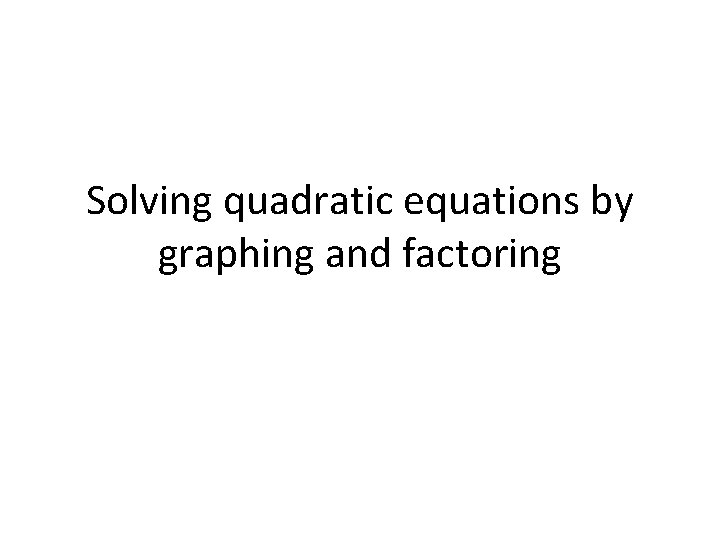Solving quadratic equations by graphing and factoringWarm Up Find the x-intercept of each function. 1. f(x) = – 3 x + 9 3 2. f(x) = 6 x + 4 Factor each expression. 3. 3 x 2 – 12 x 3 x(x – 4) 4. x 2 – 9 x + 18(x – 6)(x – 3) 5. x 2 – 49 (x – 7)(x + 7)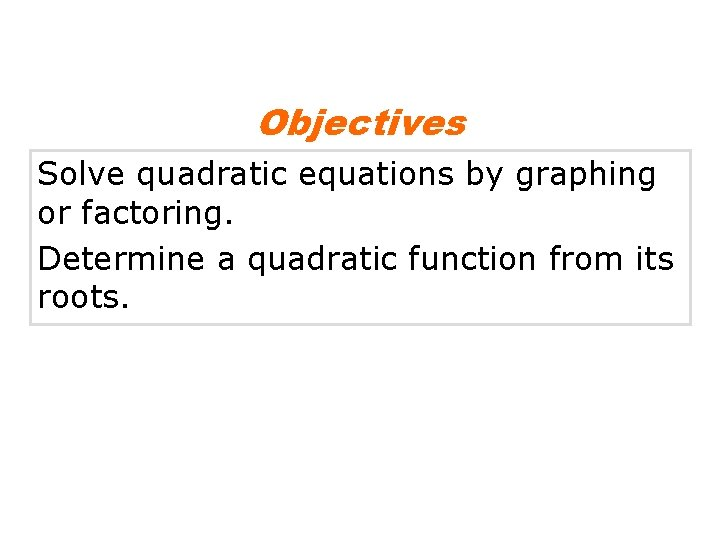Objectives Solve quadratic equations by graphing or factoring. Determine a quadratic function from its roots.Vocabulary zero of a function root of an equation binomial trinomialWhen a soccer ball is kicked into the air, how long will the ball take to hit the ground? The height h in feet of the ball after t seconds can be modeled by the quadratic function h(t) = – 16 t 2 + 32 t. In this situation, the value of the function represents the height of the soccer ball. When the ball hits the ground, the value of the function is zero.A zero of a function is a value of the input x that makes the output f(x) equal zero. The zeros of a function are the x-intercepts. Unlike linear functions, which have no more than one zero, quadratic functions can have two zeros, as shown at right. These zeros are always symmetric about the axis of symmetry.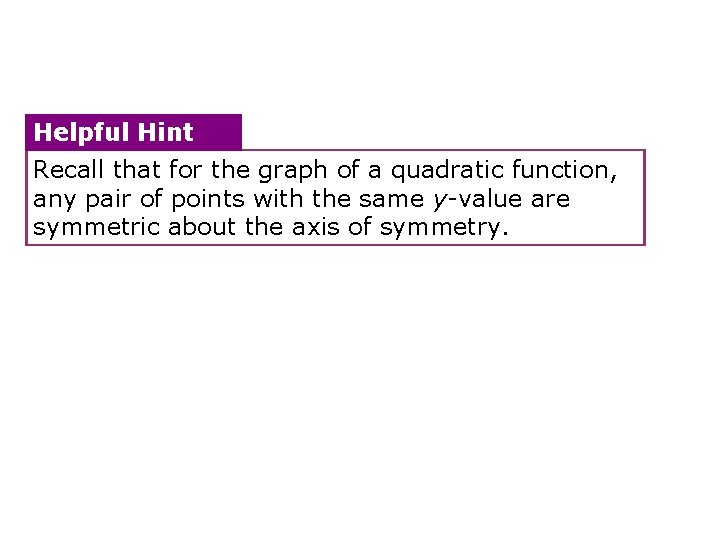Helpful Hint Recall that for the graph of a quadratic function, any pair of points with the same y-value are symmetric about the axis of symmetry.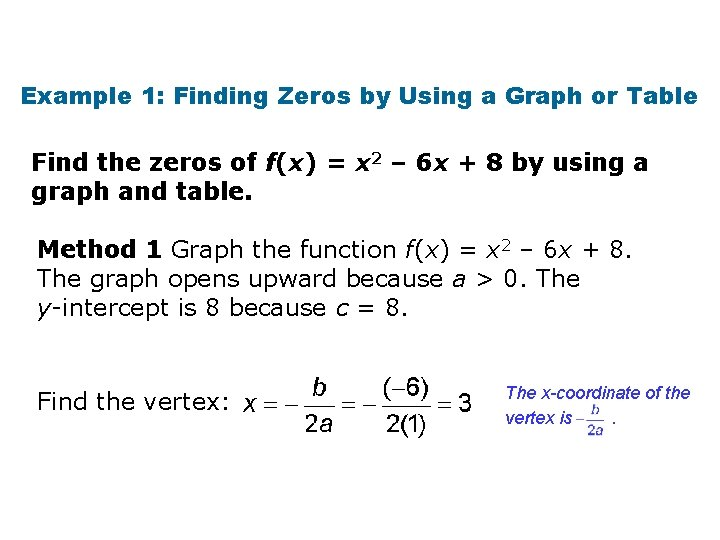Example 1: Finding Zeros by Using a Graph or Table Find the zeros of f(x) = x 2 – 6 x + 8 by using a graph and table. Method 1 Graph the function f(x) = x 2 – 6 x + 8. The graph opens upward because a > 0. The y-intercept is 8 because c = 8. Find the vertex: The x-coordinate of the vertex is.Example 1 Continued Find the zeros of f(x) = x 2 – 6 x + 8 by using a graph and table. Find f(3): f(x) = x 2 – 6 x + 8 f(3) = (3)2 – 6(3) + 8 f(3) = 9 – 18 + 8 f(3) = – 1 The vertex is (3, – 1) Substitute 3 for x.Example 1 Continued Plot the vertex and the y-intercept. Use symmetry and a table of values to find additional points. x f(x) 1 3 2 0 3 – 1 4 0 The table and the graph indicate that the zeros are 2 and 4. 5 3 (2, 0) (4, 0)Example 1 Continued Find the zeros of f(x) = x 2 – 6 x + 8 by using a graph and table. Method 2 Use a calculator. Enter y = x 2 – 6 x + 8 into a graphing calculator. Both the table and the graph show that y = 0 at x = 2 and x = 4. These are the zeros of the function.Check It Out! Example 1 Find the zeros of g(x) = –x 2 – 2 x + 3 by using a graph and a table. Method 1 Graph the function g(x) = –x 2 – 2 x + 3. The graph opens downward because a < 0. The y-intercept is 3 because c = 3. Find the vertex: The x-coordinate of the vertex is.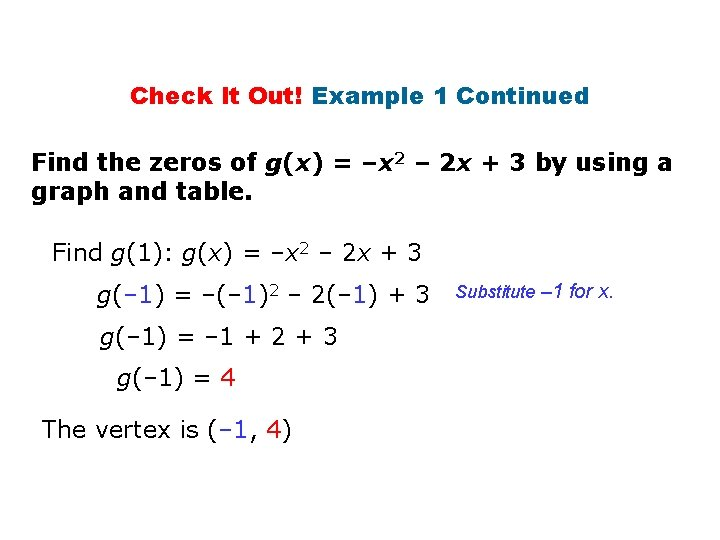Check It Out! Example 1 Continued Find the zeros of g(x) = –x 2 – 2 x + 3 by using a graph and table. Find g(1): g(x) = –x 2 – 2 x + 3 g(– 1) = –(– 1)2 – 2(– 1) + 3 g(– 1) = – 1 + 2 + 3 g(– 1) = 4 The vertex is (– 1, 4) Substitute – 1 for x.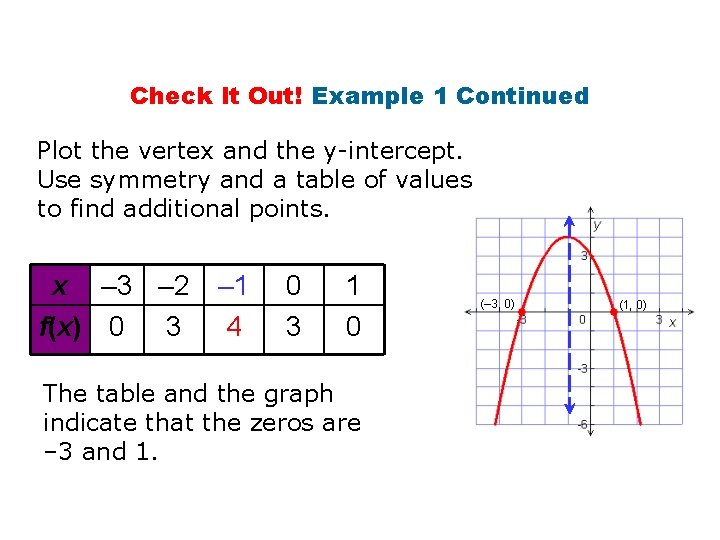Check It Out! Example 1 Continued Plot the vertex and the y-intercept. Use symmetry and a table of values to find additional points. x – 3 – 2 f(x) 0 3 – 1 4 0 3 1 0 The table and the graph indicate that the zeros are – 3 and 1. (– 3, 0) (1, 0)Check It Out! Example 1 Continued Find the zeros of f(x) = –x 2 – 2 x + 3 by using a graph and table. Method 2 Use a calculator. Enter y = –x 2 – 2 x + 3 into a graphing calculator. Both the table and the graph show that y = 0 at x = – 3 and x = 1. These are the zeros of the function.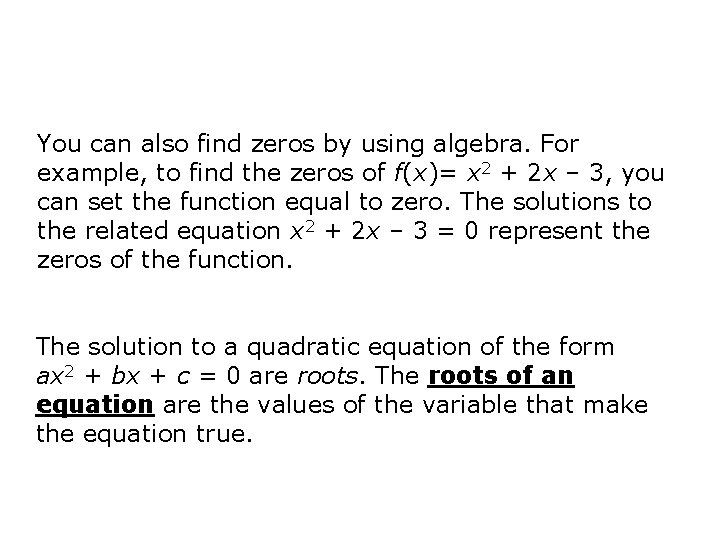You can also find zeros by using algebra. For example, to find the zeros of f(x)= x 2 + 2 x – 3, you can set the function equal to zero. The solutions to the related equation x 2 + 2 x – 3 = 0 represent the zeros of the function. The solution to a quadratic equation of the form ax 2 + bx + c = 0 are roots. The roots of an equation are the values of the variable that make the equation true.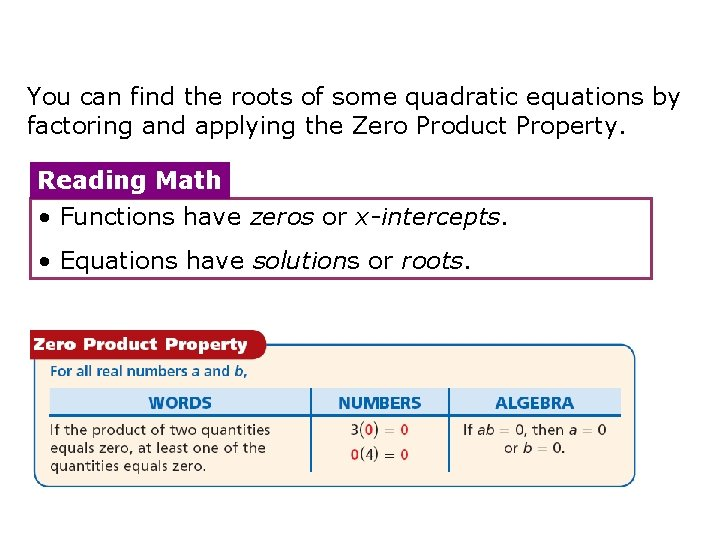You can find the roots of some quadratic equations by factoring and applying the Zero Product Property. Reading Math • Functions have zeros or x-intercepts. • Equations have solutions or roots.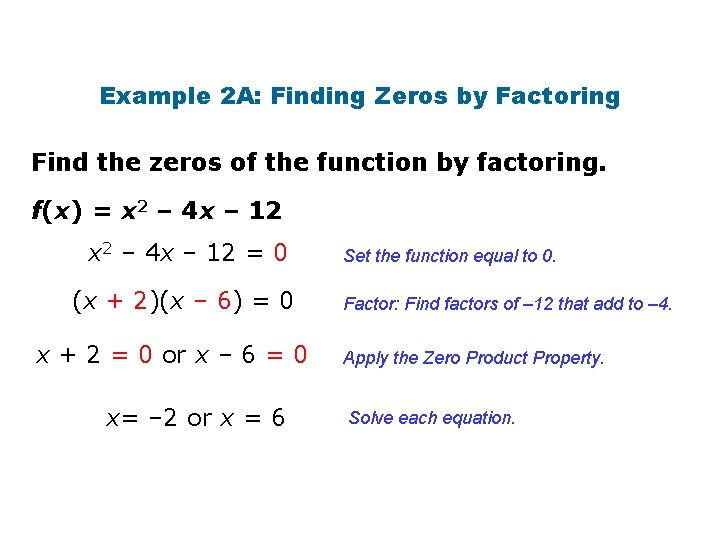Example 2 A: Finding Zeros by Factoring Find the zeros of the function by factoring. f(x) = x 2 – 4 x – 12 = 0 (x + 2)(x – 6) = 0 x + 2 = 0 or x – 6 = 0 x= – 2 or x = 6 Set the function equal to 0. Factor: Find factors of – 12 that add to – 4. Apply the Zero Product Property. Solve each equation.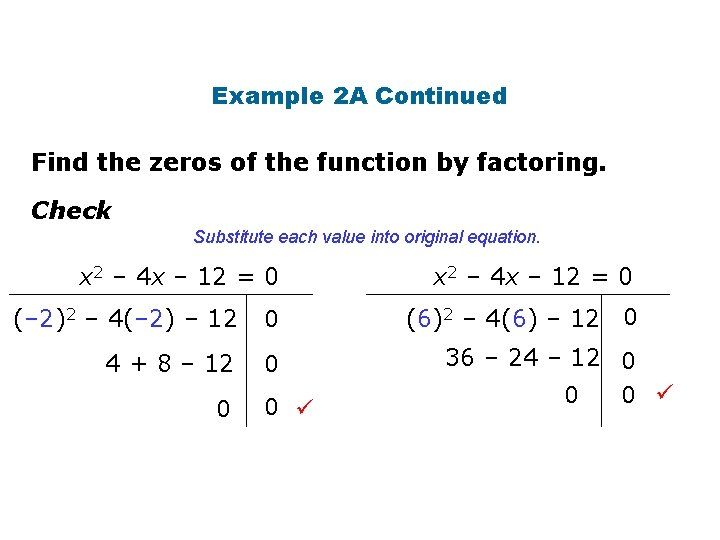Example 2 A Continued Find the zeros of the function by factoring. Check Substitute each value into original equation. x 2 – 4 x – 12 = 0 (– 2)2 – 4(– 2) – 12 0 4 + 8 – 12 0 0 0 x 2 – 4 x – 12 = 0 (6)2 – 4(6) – 12 0 36 – 24 – 12 0 0 0Example 2 B: Finding Zeros by Factoring Find the zeros of the function by factoring. g(x) = 3 x 2 + 18 x = 0 3 x(x+6) = 0 3 x = 0 or x + 6 = 0 x = 0 or x = – 6 Set the function to equal to 0. Factor: The GCF is 3 x. Apply the Zero Product Property. Solve each equation.Example 2 B Continued Check algebraically and by graphing. 3 x 2 + 18 x = 0 3(0)2 + 18(0) 0+0 0 0 3(– 6)2 + 18(– 6) 0 0 108 – 108 0 0 0 25 – 10 5 – 30 0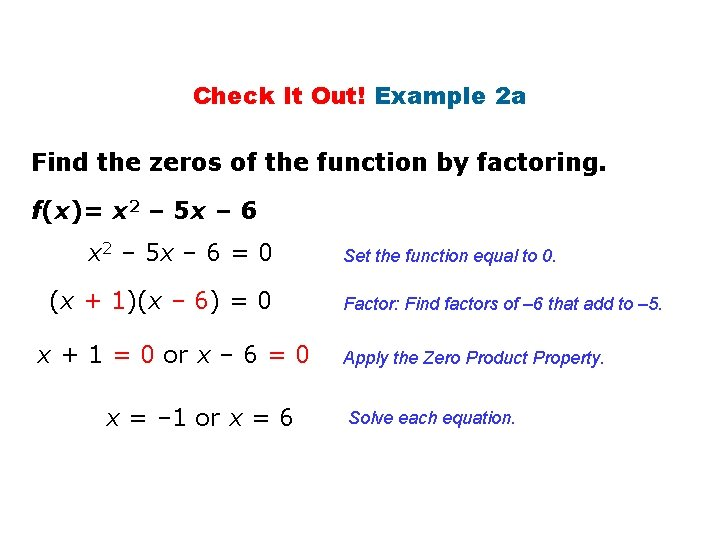Check It Out! Example 2 a Find the zeros of the function by factoring. f(x)= x 2 – 5 x – 6 = 0 (x + 1)(x – 6) = 0 x + 1 = 0 or x – 6 = 0 x = – 1 or x = 6 Set the function equal to 0. Factor: Find factors of – 6 that add to – 5. Apply the Zero Product Property. Solve each equation.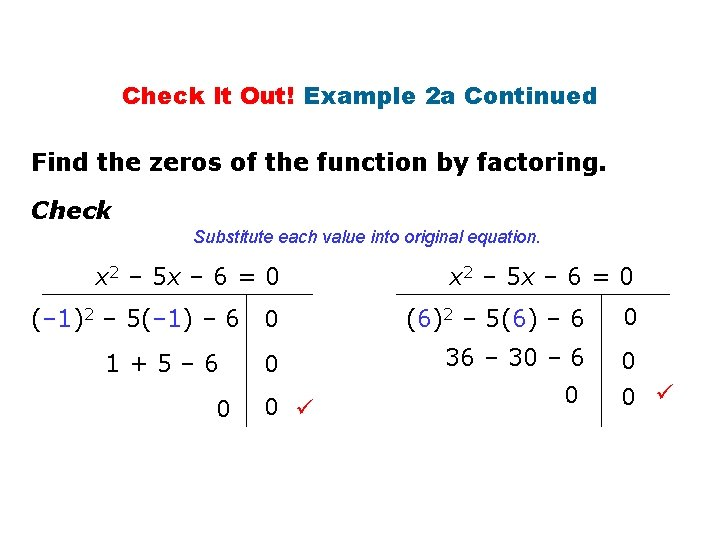Check It Out! Example 2 a Continued Find the zeros of the function by factoring. Check Substitute each value into original equation. x 2 – 5 x – 6 = 0 (– 1)2 – 5(– 1) – 6 1+5– 6 0 x 2 – 5 x – 6 = 0 0 (6)2 – 5(6) – 6 0 36 – 30 – 6 0 0 0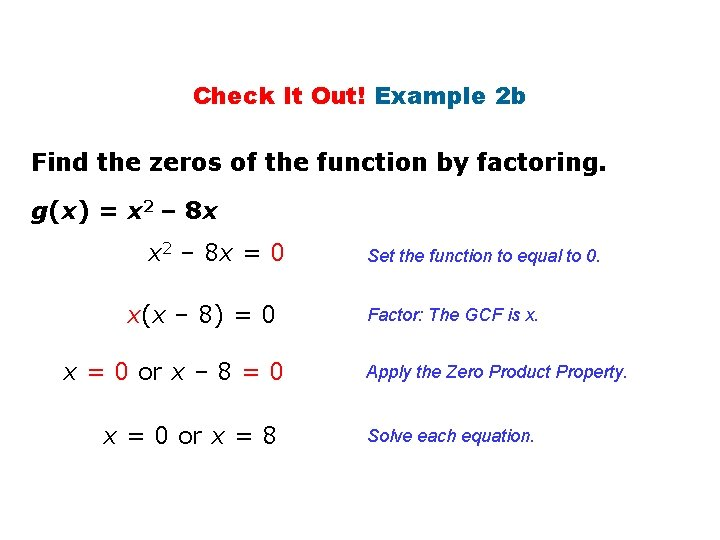Check It Out! Example 2 b Find the zeros of the function by factoring. g(x) = x 2 – 8 x = 0 x(x – 8) = 0 x = 0 or x – 8 = 0 x = 0 or x = 8 Set the function to equal to 0. Factor: The GCF is x. Apply the Zero Product Property. Solve each equation.Check It Out! Example 2 b Continued Find the zeros of the function by factoring. Check Substitute each value into original equation. x 2 – 8 x = 0 (0)2 – 8(0) 0 0– 0 0 x 2 – 8 x = 0 (8)2 – 8(8) 64 – 64 0 0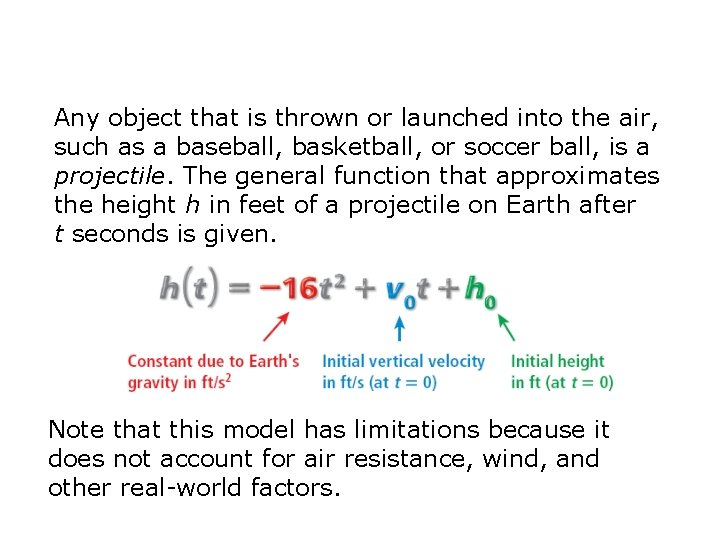Any object that is thrown or launched into the air, such as a baseball, basketball, or soccer ball, is a projectile. The general function that approximates the height h in feet of a projectile on Earth after t seconds is given. Note that this model has limitations because it does not account for air resistance, wind, and other real-world factors.Example 3: Sports Application A golf ball is hit from ground level with an initial vertical velocity of 80 ft/s. After how many seconds will the ball hit the ground? h(t) = – 16 t 2 + v 0 t + h 0 Write the general projectile function. h(t) = – 16 t 2 + 80 t + 0 Substitute 80 for v 0 and 0 for h 0.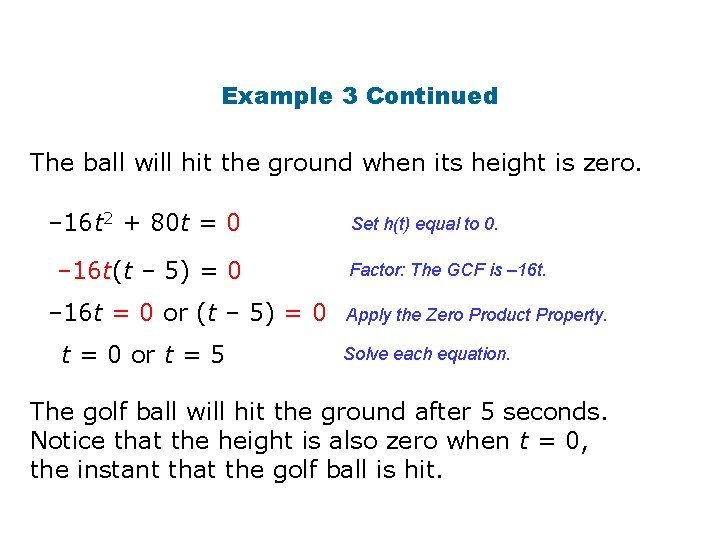Example 3 Continued The ball will hit the ground when its height is zero. – 16 t 2 + 80 t = 0 – 16 t(t – 5) = 0 – 16 t = 0 or (t – 5) = 0 t = 0 or t = 5 Set h(t) equal to 0. Factor: The GCF is – 16 t. Apply the Zero Product Property. Solve each equation. The golf ball will hit the ground after 5 seconds. Notice that the height is also zero when t = 0, the instant that the golf ball is hit.Example 3 Continued Check The graph of the function h(t) = – 16 t 2 + 80 t shows its zeros at 0 and 5. 105 – 3 7 – 15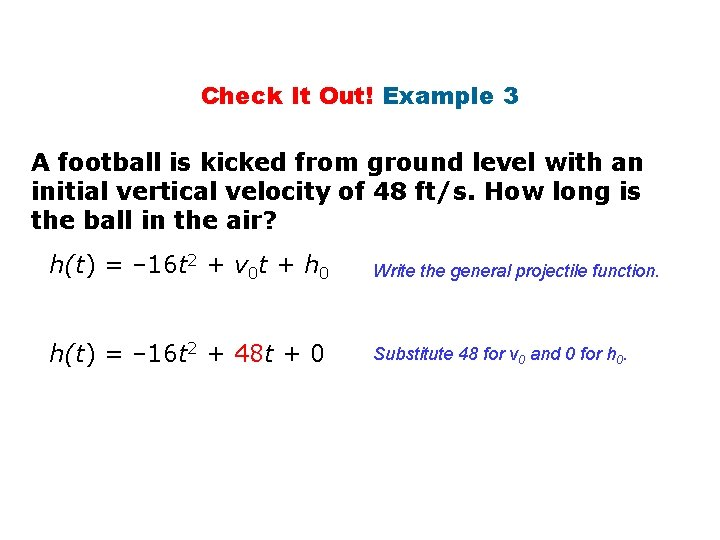Check It Out! Example 3 A football is kicked from ground level with an initial vertical velocity of 48 ft/s. How long is the ball in the air? h(t) = – 16 t 2 + v 0 t + h 0 Write the general projectile function. h(t) = – 16 t 2 + 48 t + 0 Substitute 48 for v 0 and 0 for h 0.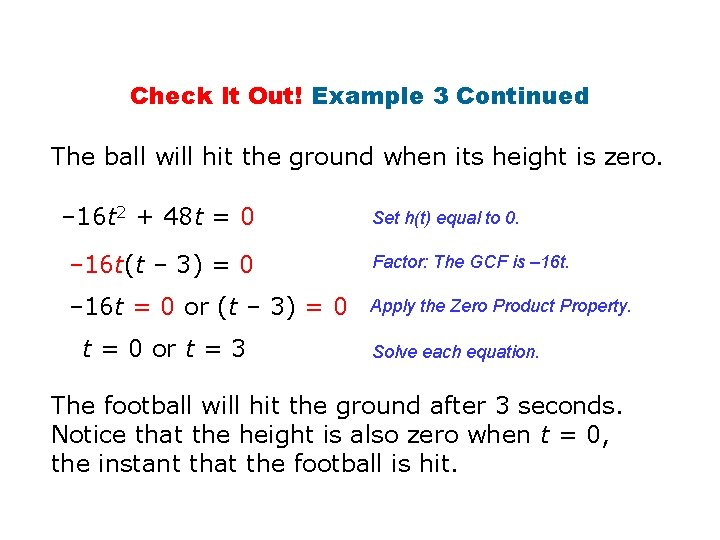Check It Out! Example 3 Continued The ball will hit the ground when its height is zero. – 16 t 2 + 48 t = 0 Set h(t) equal to 0. – 16 t(t – 3) = 0 Factor: The GCF is – 16 t = 0 or (t – 3) = 0 Apply the Zero Product Property. t = 0 or t = 3 Solve each equation. The football will hit the ground after 3 seconds. Notice that the height is also zero when t = 0, the instant that the football is hit.Check It Out! Example 3 Continued Check The graph of the function h(t) = – 16 t 2 + 48 t shows its zeros at 0 and 3. 40 – 1 5 – 15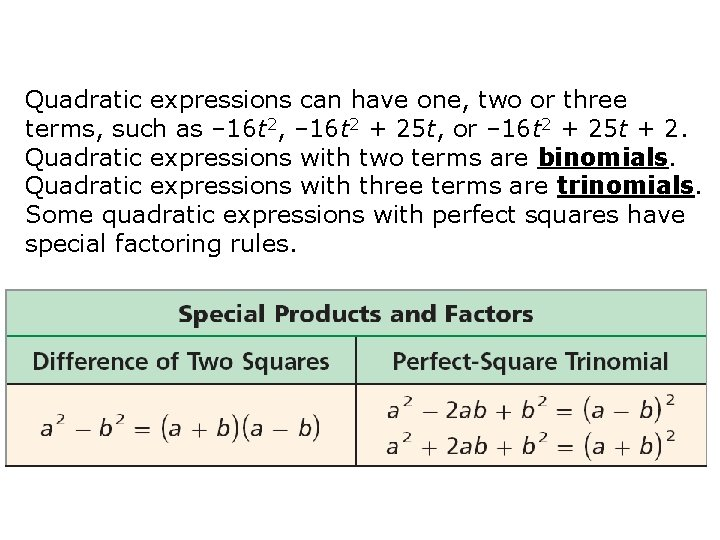Quadratic expressions can have one, two or three terms, such as – 16 t 2, – 16 t 2 + 25 t, or – 16 t 2 + 25 t + 2. Quadratic expressions with two terms are binomials. Quadratic expressions with three terms are trinomials. Some quadratic expressions with perfect squares have special factoring rules.Example 4 A: Find Roots by Using Special Factors Find the roots of the equation by factoring. 4 x 2 = 25 4 x 2 – 25 = 0 (2 x)2 – (5)2 = 0 Rewrite in standard form. Write the left side as a 2 – b 2. (2 x + 5)(2 x – 5) = 0 Factor the difference of squares. 2 x + 5 = 0 or 2 x – 5 = 0 Apply the Zero Product Property. x=– or x = Solve each equation.Example 4 Continued Check Graph each side of the equation on a graphing calculator. Let Y 1 equal 4 x 2, and let Y 2 equal 25. The graphs appear to intersect at and.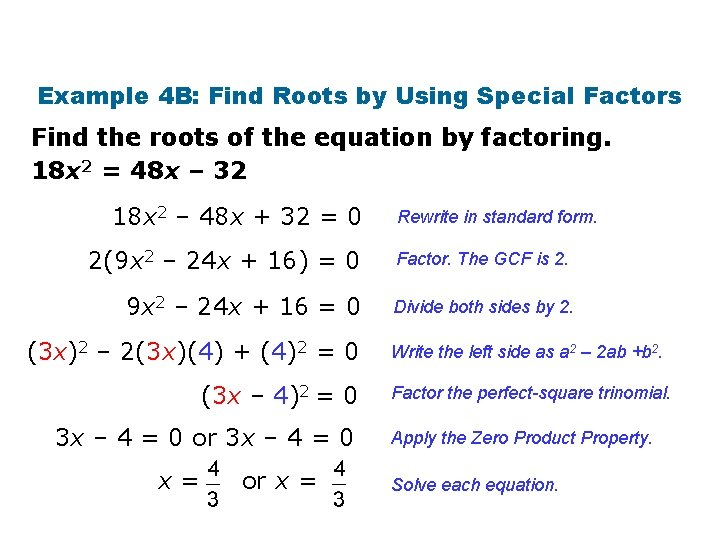Example 4 B: Find Roots by Using Special Factors Find the roots of the equation by factoring. 18 x 2 = 48 x – 32 18 x 2 – 48 x + 32 = 0 Rewrite in standard form. 2(9 x 2 – 24 x + 16) = 0 Factor. The GCF is 2. 9 x 2 – 24 x + 16 = 0 Divide both sides by 2. (3 x)2 – 2(3 x)(4) + (4)2 = 0 (3 x – 4)2 = 0 3 x – 4 = 0 or 3 x – 4 = 0 x= or x = Write the left side as a 2 – 2 ab +b 2. Factor the perfect-square trinomial. Apply the Zero Product Property. Solve each equation.Example 4 B Continued Check Substitute the root equation. into the original 18 x 2 = 48 x – 32 18 18 32 2 48 – 32 64 – 32 32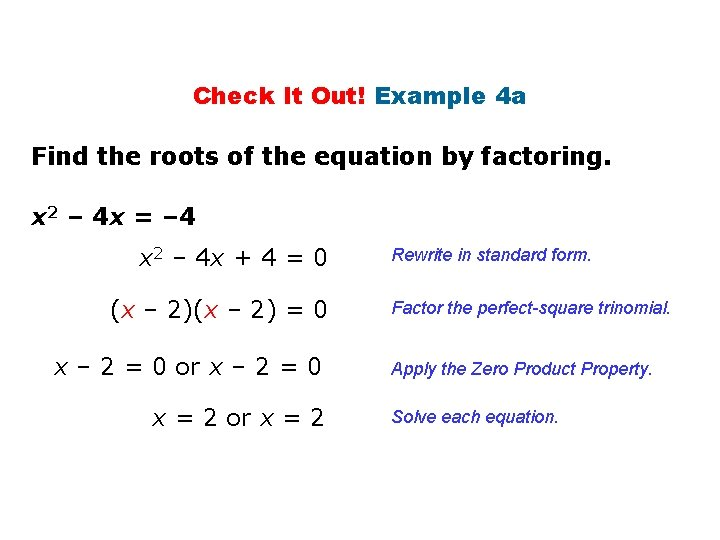Check It Out! Example 4 a Find the roots of the equation by factoring. x 2 – 4 x = – 4 x 2 – 4 x + 4 = 0 (x – 2) = 0 x – 2 = 0 or x – 2 = 0 x = 2 or x = 2 Rewrite in standard form. Factor the perfect-square trinomial. Apply the Zero Product Property. Solve each equation.Check It Out! Example 4 a Continued Check Substitute the root 2 into the original equation. x 2 – 4 x = – 4 (2)2 – 4(2) – 4 4 – 8 – 4 – 4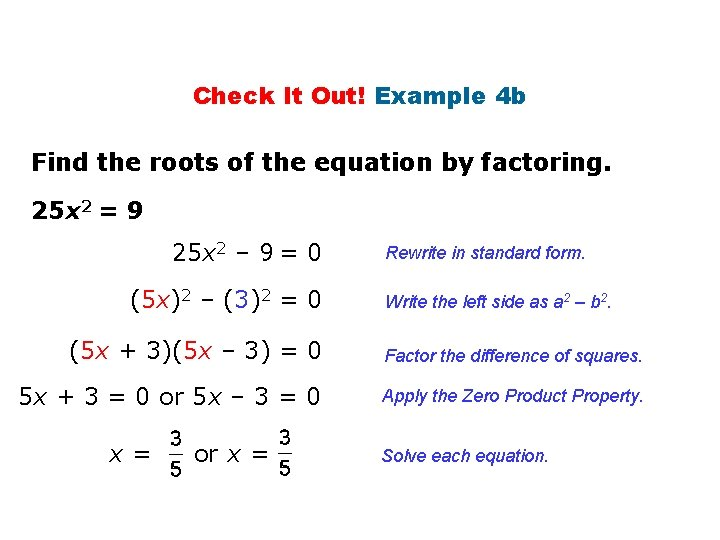Check It Out! Example 4 b Find the roots of the equation by factoring. 25 x 2 = 9 25 x 2 – 9 = 0 (5 x)2 – (3)2 = 0 Rewrite in standard form. Write the left side as a 2 – b 2. (5 x + 3)(5 x – 3) = 0 Factor the difference of squares. 5 x + 3 = 0 or 5 x – 3 = 0 Apply the Zero Product Property. x= or x = Solve each equation.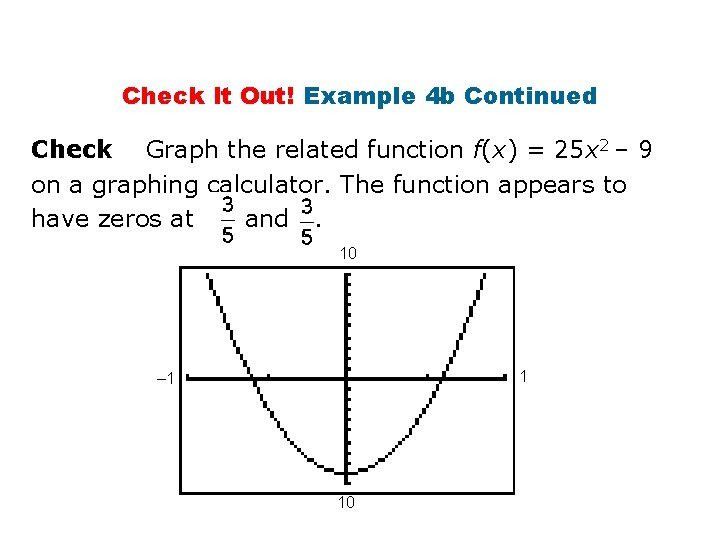Check It Out! Example 4 b Continued Check Graph the related function f(x) = 25 x 2 – 9 on a graphing calculator. The function appears to have zeros at and. 10 1 – 1 10If you know the zeros of a function, you can work backward to write a rule for the function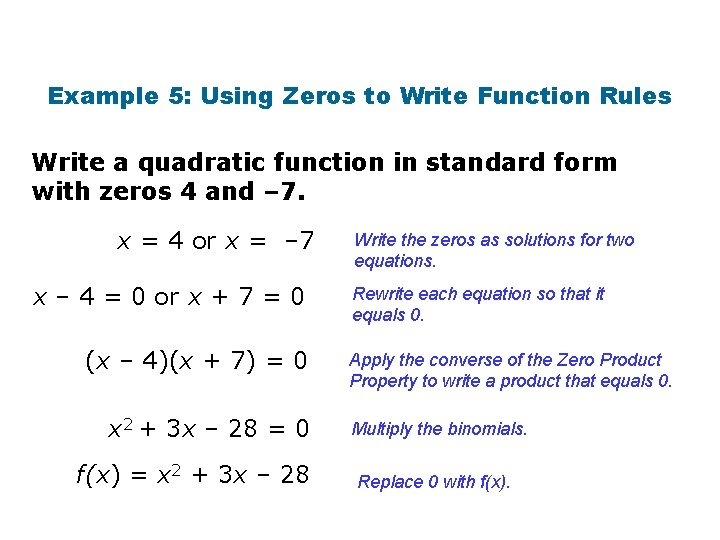Example 5: Using Zeros to Write Function Rules Write a quadratic function in standard form with zeros 4 and – 7. x = 4 or x = – 7 x – 4 = 0 or x + 7 = 0 (x – 4)(x + 7) = 0 x 2 + 3 x – 28 = 0 f(x) = x 2 + 3 x – 28 Write the zeros as solutions for two equations. Rewrite each equation so that it equals 0. Apply the converse of the Zero Product Property to write a product that equals 0. Multiply the binomials. Replace 0 with f(x).Example 5 Continued Check Graph the function f(x) = x 2 + 3 x – 28 on a calculator. The graph shows the original zeros of 4 and – 7. 10 10 – 35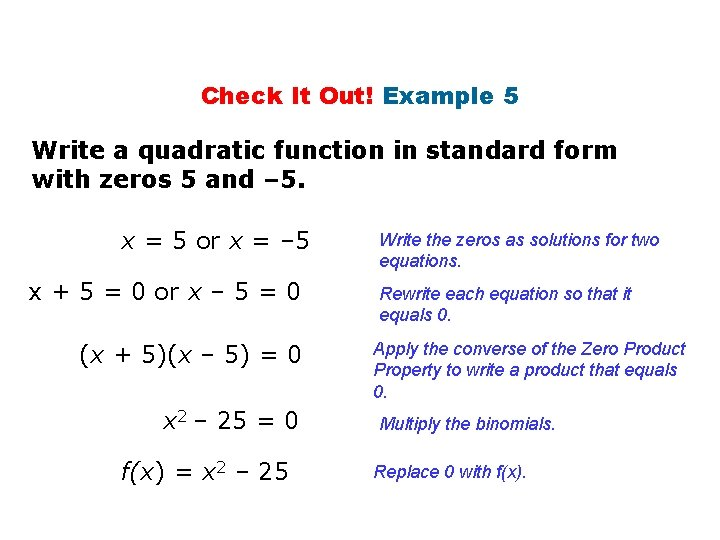Check It Out! Example 5 Write a quadratic function in standard form with zeros 5 and – 5. x = 5 or x = – 5 x + 5 = 0 or x – 5 = 0 (x + 5)(x – 5) = 0 x 2 – 25 = 0 f(x) = x 2 – 25 Write the zeros as solutions for two equations. Rewrite each equation so that it equals 0. Apply the converse of the Zero Product Property to write a product that equals 0. Multiply the binomials. Replace 0 with f(x).Check It Out! Example 5 Continued 10 Check Graph the function f(x) = x 2 – 25 on a calculator. The graph shows the original zeros of 5 and – 5. – 8 8 – 30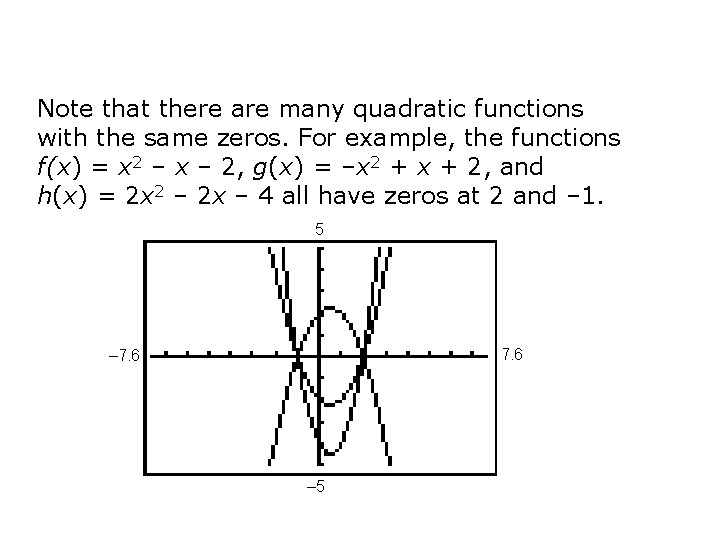Note that there are many quadratic functions with the same zeros. For example, the functions f(x) = x 2 – x – 2, g(x) = –x 2 + x + 2, and h(x) = 2 x 2 – 2 x – 4 all have zeros at 2 and – 1. 5 7. 6 – 5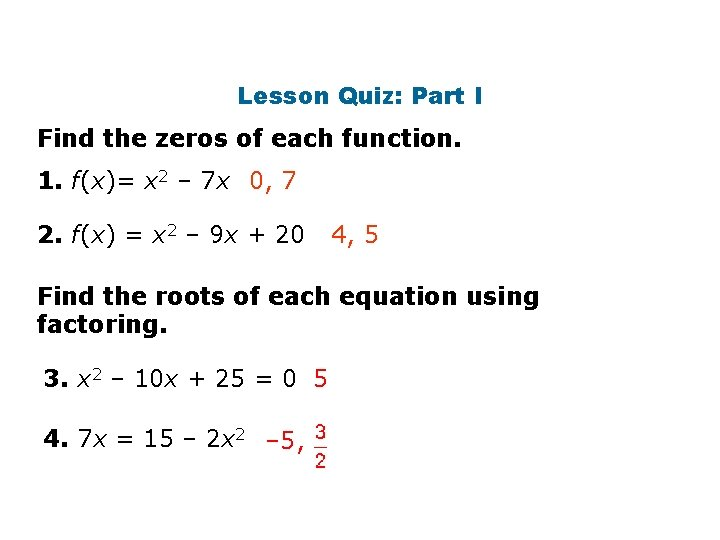Lesson Quiz: Part I Find the zeros of each function. 1. f(x)= x 2 – 7 x 0, 7 2. f(x) = x 2 – 9 x + 20 4, 5 Find the roots of each equation using factoring. 3. x 2 – 10 x + 25 = 0 5 4. 7 x = 15 – 2 x 2 – 5,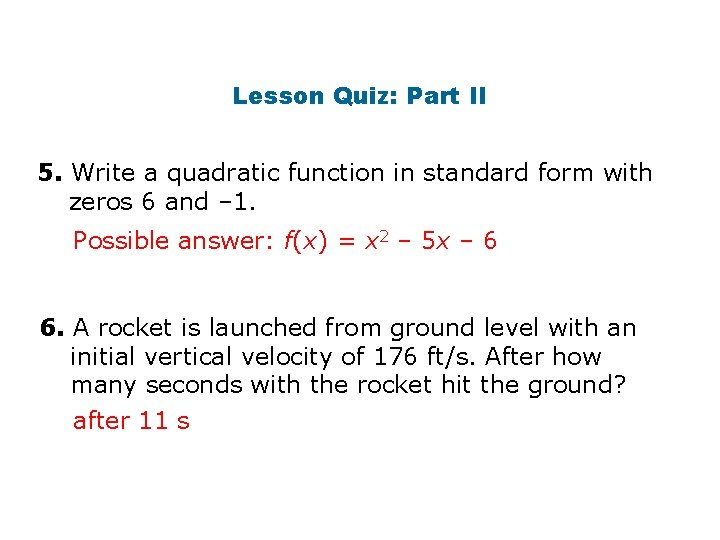Lesson Quiz: Part II 5. Write a quadratic function in standard form with zeros 6 and – 1. Possible answer: f(x) = x 2 – 5 x – 6 6. A rocket is launched from ground level with an initial vertical velocity of 176 ft/s. After how many seconds with the rocket hit the ground? after 11 s